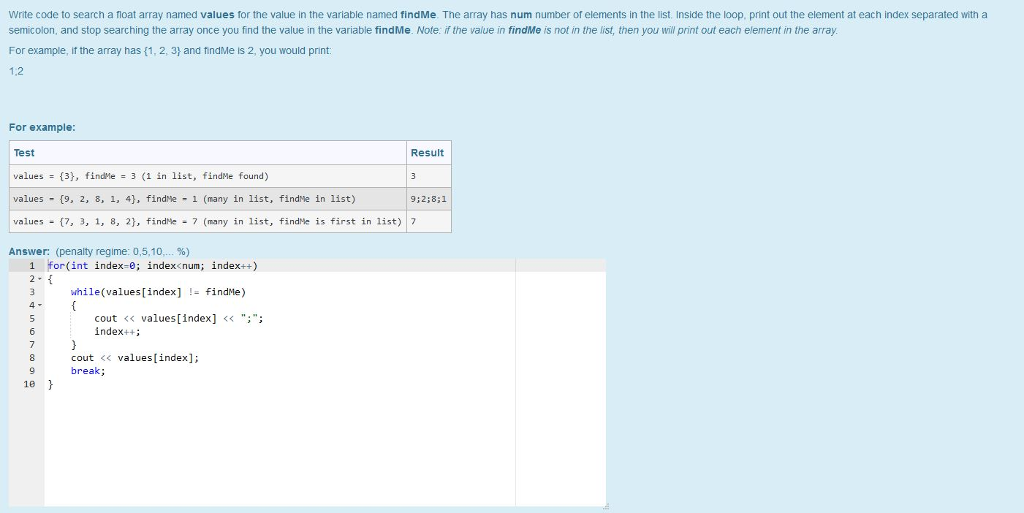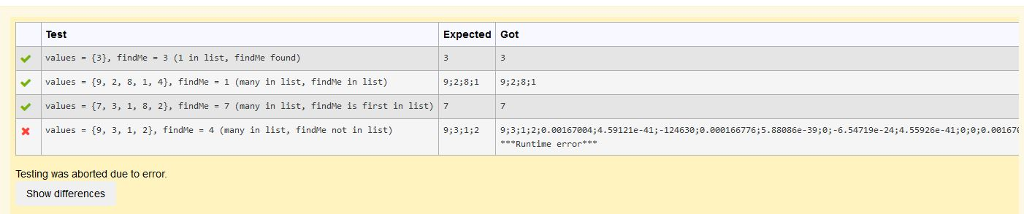# Question & Answer: Write code to search a foat array named values for the value in the variable named findMe. The…..

C++ Problem:

I can’t figure out why I am not passing the last test case in CodeRunner. Any help is appreciated, especially if you can solve the problem.

Don't use plagiarized sources. Get Your Custom Essay on
Question & Answer: Write code to search a foat array named values for the value in the variable named findMe. The…..
GET AN ESSAY WRITTEN FOR YOU FROM AS LOW AS \$13/PAGETest Cases:Write code to search a foat array named values for the value in the variable named findMe. The array has num number of elements in the list. Inside the loop. print out the element at each index separated with a semicolon, and stop searching the array once you find the value in the variable findMe. Note: if the value in findMe is not in the list, then you will print out each element in the array. For example, it the array has (1, 2, 31 and findMe is 2, you would print 1.2 For example Test values = {3}, findMe = 3 (1 in list, findMe found) Result values- (9, 2, 8, 1, 4), findMe-1 (many in list, findMe in list) values _ {7, 3, 1, 8, 2), findHe-7 (many in list, findse is first in list)17 Answer: (penalty regime: 05.10 %) for (int index-e; index

Because when the element is not present , index is going out of Bound

for (int index =0; index<num ; ++index){

while(index < num && values[index] != findMe){
if(index==num-1){
cout<<values[index];

}else{
cout<<values[index] << “; “;

}
++index;

}
if(index<num){
cout<<values[index];
}

break;

}